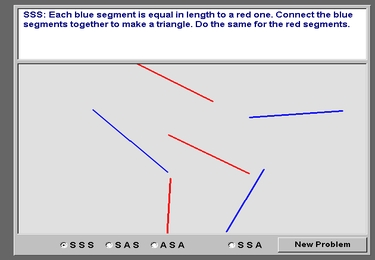## Geometry

PIN number: FSOVXH

1.### L6555 Exploring angles

• Published 01/03/2016
• TLF-ID L6555

Resize a triangle by moving its vertices. Notice that the sum of the three interior angles is always 180 degrees. Watch a video showing how angles are used in designing and riding bicycles.

2.### L6558 Exploring triangles

• Published 04/03/2016
• TLF-ID L6558

Find an active triangle in a photograph. Work out its angles by applying principles of opposite angles, complementary angles, supplementary angles and the sum of interior angles. Watch a video showing how triangles are used in buildings and other structures.

3.### L6554 Exploring relationships of angles

• Published 05/04/2016
• TLF-ID L6554

Explore angles formed by a transversal line intersecting parallel lines. Look at illustrations showing pairs of angles: vertically opposite, corresponding and alternate angles. Name pairs of angles to score points and help a monkey drive to the supermarket to buy food.

4.• Published 01/03/2016
• TLF-ID L6562

Examine the sides and angles of a four-sided shape. Identify its geometric properties such as the number of sides of equal length. Classify the shape as a parallelogram, rhombus, square, rectangle, kite or trapezium. Notice that some quadrilaterals can be classified in different ways.

5.### L2327 Trigonometry: similar triangles

• Published 10/04/2020
• TLF-ID L2327

Examine pairs of right-angled triangles. Check that each pair of triangles is similar by identifying all three pairs of corresponding angles. Label corresponding angles with matching symbols. This learning object is one in a series of ten objects.

6.### L3517 Congruent triangles

• Published 24/01/2014
• TLF-ID L3517

Find out about congruent and non-congruent triangles and the conditions required to make them. Use line segments and angles to build two congruent triangles for three different combinations of sides and angles. Explore the SSS case (side, side, side), the SAS case (two sides and the included angle) and the ASA case (two angles and the included side) by forming two triangles and then using the rotation function to test them for congruency. Examine the SSA condition (two sides and an angle opposite one of them, often called the Ambiguous Case), and determine the conditions needed to form non-congruent triangles. This resource requires the current Java Plug-in to be installed.

7.